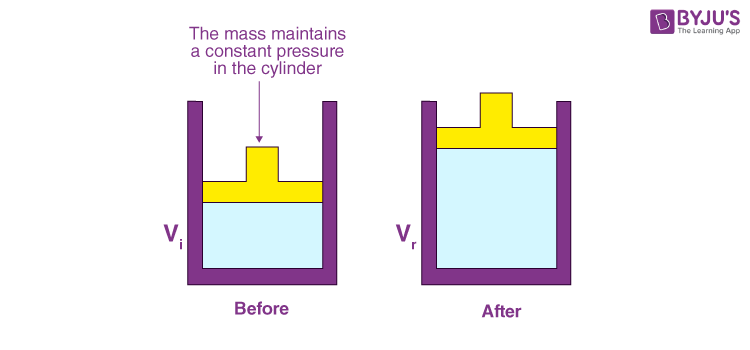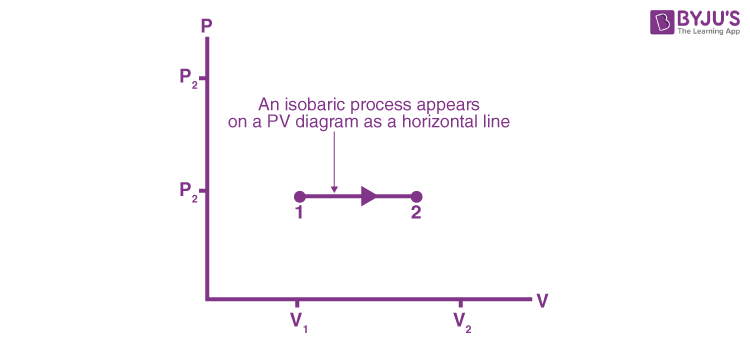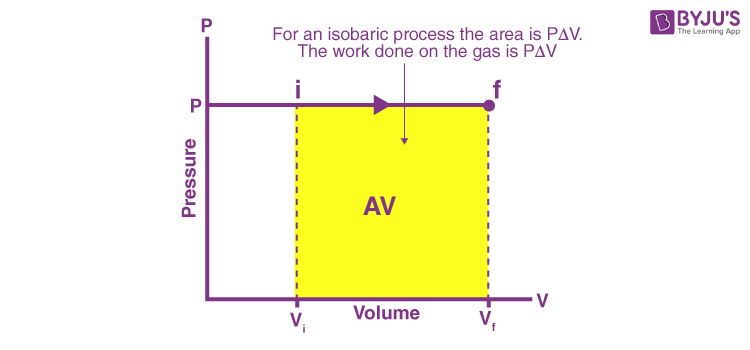# Isobaric Process

## What is Isobaric Process?

An Isobaric process is a thermodynamic process taking place at constant pressure. The term isobaric has been derived from the Greek words “iso” and “baros” meaning equal pressure. As such, the constant pressure is obtained when the volume is expanded or contracted. This basically neutralizes any pressure change due to the transfer of heat.

In an isobaric process, when the heat is transferred to the system some work is done. However, there is also a change in the internal energy of the system. This further means that no quantities as in the first law of thermodynamics become zero.

## Constant Pressure Process

An isobaric process is  also called constant pressure process.

${{P}_{f}}={{P}_{i}}\,\,\,\left( \Delta P=0\,\,and\,\,dP=0 \right)$

The figure below shows one method of changing the state of gas while keeping the pressure constant.A cylinder of gas has a tight-fitting, massless piston that can slide up and down but the container is closed so that no atoms enter or escape. The mass on top applies a constant downward force Mg on the piston. The atmosphere also presses down on the piston. In equilibrium, the upward force PA on the piston by the gas in the cylinder, where A is the piston’s area, exactly balances the downward force Mg plus the air-pressure force Patmos A. Consequently, the gas pressure inside the cylinder is

$P={{P}_{\text{atmos}}}+\frac{Mg}{A}=\text{constant}$

This pressure is independent of the temperature of the gas or the height of the piston, so it stays constant as long as M is unchanged.

If the cylinder is warmed, the gas will expand and push the piston up. But the pressure, determined by mass M, will not change. This process is shown on the P – V diagram as the horizontal line 1 → 2. We call this an isobaric expansion. As isobaric compression occurs if the gas is cooled, lowering the piston. An isobaric process appears on a P – V diagram as a horizontal line.## Isobaric Process Example

An example of the isobaric process includes the boiling of water to steam or the freezing of water to ice. In the process, a gas either expands or contracts to maintain constant pressure and hence the net amount of work is done by the system or on the system. The amount of heat dQ is partly used in increasing the temperature dT and partly used in doing external work.

$dQ={{C}_{P}}dT+P\,\,dV$

## Work Done by a Gas in an Isobaric Process

The work done is found from the equation;

$W=\int_{{{V}_{i}}}^{{{V}_{f}}}{P\,dV}$Since the pressure is constant in an isobaric process, the integral becomes

$W={{P}_{i}}\int_{{{V}_{i}}}^{{{V}_{f}}}{dV\,={{P}_{i}}}\left( {{V}_{f}}-{{V}_{i}} \right)$ $={{P}_{i}}\,\Delta V$

If the gas expands, ${{V}_{f}}>{{V}_{i}},$ so ΔV > 0 and the work done by the gas is positive.

When the gas is compressed, ${{V}_{f}}<{{V}_{i}}$ so that ΔV < 0, and the work that is done by the gas is negative.

The work done by the gas is represented on the P-V diagram by the rectangular area under the isobaric path on the diagram. Whether the area is positive or negative, depends on whether the gas expands or is compressed.

## What is Thermodynamic Process?

A process occurs when the system changes from one state (one set of values of its physical properties) to another state. The system returns to its original state when all of its macroscopic physical properties resume their original values.

Heat transfer and work are two processes that change the state of thermodynamic equilibrium. A quasi-static process is one in which the system changes slowly enough so that each succeeding state through which it passes is essentially in equilibrium. All the reversible processes are quasi-static (occur very slowly). An equilibrium state is a static state. During a reversible process, the system can deviate from equilibrium by only an infinitesimal amount.

There are other thermodynamic processes in equilibrium thermodynamics. The processes are characterized by the thermodynamic variable that is kept constant.

• Isothermal process: A process is isothermal when the temperature of the system is kept constant.
• Isochoric process: A process is isochoric when the volume of the system is constant.
• Adiabatic process: A process is adiabatic when no heat is transferred to or from the system.